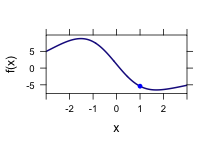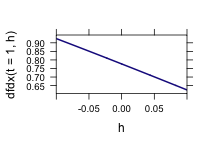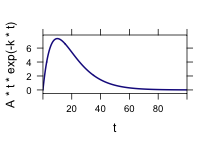# Modeling with Limits

Danny Kaplan

Judging from college catalog descriptions of calculus courses, limits and series are a standard part of the first-year college calculus curriculum. In informal surveys of mathematics instructors, they are identified as central components of calculus, along with derivatives and integrals.

In teaching calculus in a modeling-based way, it's worth thinking about why limits and series are included. Of course there are benefits to teaching about limits and series — more knowledge is better! — but there are also costs. In particular, the emphasis on limits and infinite series can divert students
from important modeling concepts such a “big” and “small” and “close.”

The mathematical approach to “big” and “small” corresponds to $$\lim_{x\rightarrow \infty}$$ and $$\lim_{x\rightarrow 0}$$, but in modeling it's almost always necessary to talk about big and small compared to something else. The mathematical take on series is about convergence, whereas in modeling it's about approximation. Insofar as calculus is taught as an approach to exactitude and equality, it may interfere with the development of an understanding of approximation, “good enough”, and the relevant physical domain of a model. Traditionally, calculus pushes things to the limit, but in practice you may need “small, but not too small.”

Despite these pitfalls when modeling is a goal, teaching about limits and infinite series may have advantages.

• As a technique used in modeling and applications
• As a prelude to teaching other techniques, such as integrals and derivatives

## Limits

I'll stipulate that there are no applications of limits that are general enough to make them worthwhile to teach as a modeling technique. One way to back up such a statement is to ask non-mathematics colleagues how they use limits in their work. The typical answer will be, “I don't.” But it's hard to prove a negative of this sort, so I invite dissent and examples of limits in modeling.

It's risky to assume that teaching about limits in the traditional way will somehow translate into a better student understanding of modeling; as mentioned above, there are important ways in which the mathematical concept of limits conflicts with modeling. Nonetheless, even if limits aren't used directly in modeling, they may support understanding concepts and techniques that are important to modeling.

In strategizing about how and why to teach limits in calculus, it is helpful to distinguish between two sorts of limits: limits in the middle and limits at infinity

## Limits in the middle

Calculus textbooks often show functions with point holes in the domain, as in the picture:The main instance of this is a derivative:
$\frac{df}{dx} \approx \frac{f(x+h)-f(x)}{h} .$
It's rare to find modeling functions that have a hole in the domain, and $$df/dx$$ does not generally have a hole when considered as a function of $$x$$. It's as a function of $$h$$ that $$df/dx$$ has a hole: the division by $$h$$ means that when $$h=0$$ the function is undefined by virtue of division
by zero.

To illustrate, consider the derivative of $$A \cos(\frac{2\pi}{p} t)$$ at $$t=1$$ with some specific values of the parameters $$A$$ and $$p$$, say, $$A=2$$ and $$p=5$$:

f = makeFun(A * sin(2 * pi * t/p) ~ t, A = 2, p = 5)
dfdx = makeFun((f(t + h) - f(t))/h ~ t)
plotFun(dfdx(t = 1, h) ~ h, h.lim = range(-0.1, 0.1), lwd = 2)Where's the hole in the graph? We know the function $$df/dx$$ is undefined when $$h=0$$, but the graph doesn't show it.

It's not that the plotting software knows about limits and has done the appropriate calculation of the limit. The computer recognizes that the ratio being plotted is undefined for $$h=0$$ and will return an indication of this.

dfdx(t = 1, h = 0)

##  NaN


The plotting software is arranged so that it ignores points at which NaN is the value. Drawing a simple linear interpolation between the points at which the function is defined gives a sensible read-out of the function. Picking any small value of $$h$$ will give a very close approximation to the derivative:

fprime = D(f(t) ~ t)
fprime(t = 1)

##  0.7766

dfdx(t = 1, h = 1e-04)

##  0.7765

dfdx(t = 1, h = 1e-06)

##  0.7766

dfdx(t = 1, h = 1e-08)

##  0.7766


Since we can't define the derivative using $$h=0$$, we need to choose some other value of $$h$$. There are aesthetic reasons for choosing to define the derivative as a limit $$h \rightarrow 0$$ — you would be rightly repulsed at the idea of defining a basic concept like the derivative with a specific, non-zero $$h$$, for instance, $$h=0.000001$$.

But the practical reason to define the derivative in terms of the limit $$h \rightarrow 0$$ is to be able to leave $$h$$ out of the conversation entirely. By using the limit, we get the advantages of making $$h$$ small without the need to define what “small”“ means.

From a modeling perspective, the meaning of "small” is important and we should engage it directly when teaching about derivatives. Why?
*Often, the purpose for which a derivative is used is to indicate the rate of change of a quantity — how the output changes as the input is changed. In practice, the input will always be changed by a finite amount and so the output will change accordingly. Using the derivative defined in terms of the limit will give only an approximation to the change over a necessarily finite change in the input.
*Measurements of derivatives are often made from discrete measurements. How fast does the grass grow? The sensible thing is to measure the length one day, then come back the next day. Would you ever want to measure the length one instant and then measure it again after one second or one millisecond, etc? Each measurement invariably has error. The derivative you measure is really
$\widehat{df/dt} = f(t+h)+\epsilon_1 - (f(t)+\epsilon_2)/h$
where $$\epsilon_1$$ and $$\epsilon_2$$ are random errors. Re-arranging shows the problem:
$\widehat{df/dt} = \frac{f(t+h)-f(t)}{h} + \frac{\epsilon_1-\epsilon_2}{h}.$

In practice, $$\epsilon_1 - \epsilon_2 \neq 0$$. Making $$h$$ big helps to reduce the influence of measurement error on the result, even if it introduces a systematic bias into the value of $$\frac{f(t+h)-f(t)}{h}$$ compared to the theoretical derivative based on the limit.

In the context of computer arithmetic, the errors of rounding in floating point calculations introduce non-zero $$\epsilon_1$$ and $$\epsilon_2$$. The result is that numerical calculations of finite-difference derivatives are done with an $$h$$ of moderate size: proportional to $$\sqrt{|\epsilon_1|}$$.

In the context of measurements made in the field or lab, there are additional sources of error. These argue for a finite $$h$$. Why emphasize the limit to the exclusion of teaching some sensible about defining “small”.

* Body temperature varies slowly and systematically by about $$\pm 1$$ F over the course of the day: a so-called “circadian rhythm.” Suppose you wanted to measure the rate of change of body temperature at 6am. What factors would influence your choice of $$h$$? ANS: Precision of the thermometer. Typically, you can measure body temperature to a precision of about 0.1 F. So in choosing $$h$$ you would want to have a time interval over which the change in body temperature is bigger than 0.1 F.

Separating the measurements by a good span of time serves to make the influence of the error smaller.

### Limits at Infinity

One of the principles learned by many, perhaps most, students is that “you can't divide by zero.” Yet from time to time one is forced to deal with an expression that involves division by a quantity that might be zero. In the case of derivatives, the quantity is the step $$h$$. The notation $$h \rightarrow 0$$ reminds the user to make $$h$$ small but to make sure $$h\neq 0$$.

The algebraic techniques undercut this message of “small but not zero.” The basic algebraic process is to manipulate the expression symbolically, treating $$h$$ as if it were not zero (to avoid violating the can't-divide-by-zero principle). Then, when one finally arrives at an expression that doesn't involve any division by $$h$$, feel free to plug in zero.

With respect to derivatives, the student will soon move on to the symbolic transformations that implement differentiation and can forget all about limits. The facts that will remain are rules like $$x^2 \rightarrow 2 x$$ and “a derivative is a slope or tangent” (sic).

But there are times when division by zero occurs. For instance, in September 1997, a crew member on a US Navy missile cruiser, the USS Yorkdown, entered a zero into a database field.ref) Subsequently, this value was used as the denominator in a division calculation. The divide by zero produced a machine error which cascaded into the failure of a computer network on the ship. This caused the ship's propulsion to fail. The Yorktown was dead in the water for 2 hours and 45 minutes.

Computer engineers have overcome the objections of third-grade teachers and recognized that division by zero is not really an error but a situation that needs to be acknowledged. The IEEE floating point standard, the arithmetic standard used on most computers today, treats division by zero as an “exception” not an error; a valid result is returned.

1/0

##  Inf


You can do arithmetic with Inf just as if it were a number.

1/Inf

##  0

3 + Inf

##  Inf

-4 * Inf

##  -Inf


There are indeterminate cases that are treated sensibly by the standard, signalled by a value of NaN — “not a number”.

0 * Inf

##  NaN

Inf - Inf

##  NaN

Inf/Inf

##  NaN

0/0

##  NaN


Since such arithmetic is an element of the technical life of our students, something they can and will invoke often in their quantitative studies, perhaps a good application for limits is to explain why it makes sense to set up the rules this way.

There are other times when multiplication by infinity needs to be considered. For instance, one form of “surge” function is $$f(t) = A t \exp(-kt)$$. In the long run, as $$t$$ becomes large, what will happen to this function? It is a product of two functions: $$t$$ increases steadily while $$\exp(-kt)$$ goes to zero as $$t$$ increases. The simple arithmetic rules are indeterminate:

t = Inf
t * exp(-t)

##  NaN


A plot resolves the problem:

plotFun(A * t * exp(-k * t) ~ t, t.lim = c(0, 100), k = 0.1, A = 2)The function goes to zero on a time scale that's determined by $$k$$, not $$A$$. Perhaps even introduce an interactive script to allow students to vary the parameters and see which ones are important.

require(manipulate)
manipulate(plotFun(A * t * exp(-k * t) ~ t, t.lim = c(0, 10^n), k = k,
A = A, npts = 10000), n = slider(0, 5, step = 0.1, initial = 2, label = "Set t-max"),
k = slider(0, 0.2, step = 0.001, initial = 0.1), A = slider(-100, 100, step = 1,
initial = 3))


The traditional way to show that the surge function goes to zero as $$t \rightarrow \infty$$ is by l'Hospital's rule. First published in 1696, the Rule seems mainly to provide a mechanism, a pseudo-application, for having students take derivatives of a variety of functions and spotting singularities, places where the input to a function would cause a violation of the no-division-by-zero rule. Whether this is a help to students or a distraction is a matter of dispute, but the textbook cases are appropriately handled by the rules for computer arithmetic and the conventions of the plotting program.

#### Exercises: Functions at zero and infinity

• $$\lim_{x \rightarrow 1} \frac{\ln x}{x-1}$$
• $$\lim_{x \rightarrow 1} \frac{x^2-1}{2*x^2+1}$$
• $$\lim_{x \rightarrow \infty} \frac{e^x}{x^2}$$
• $$\lim_{x \rightarrow \infty} \frac{\ln x}{\sqrt{x}}$$
• $$\lim_{x \rightarrow 0^+} \frac{x}{1/\ln x}$$
• A counter-example from Stewart where l'Hospital's rule shouldn't be applied: $$\lim_{x \right \pi^-} \frac{sin x}{1 - \cos x}$$

Show that in each of these cases, the computer arithmetic results in NaN at the singularity, but the plot shows the limit reasonably.

#### Summary: Teaching limits in a modeling-based way:

• When introducing the derivative, $$h \rightarrow 0$$ means that $$h$$ should be small. Discuss what “small” means and how it depends on context. Two important contexts: the length scale over which the function shows visible curvature; the amount of measurement (or round-off) noise in an evaluation of the function.
• The idea that division by zero is prohibited and how the computer deals with a division by zero. Quantities that make sense near zero (or near infinity) are reasonably interpreted in the limit.